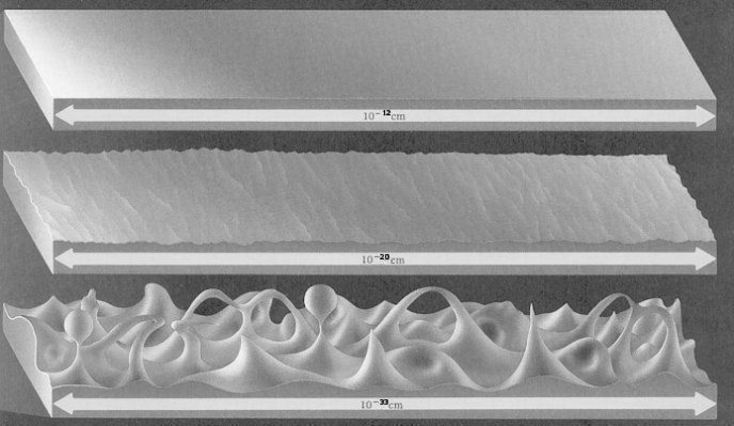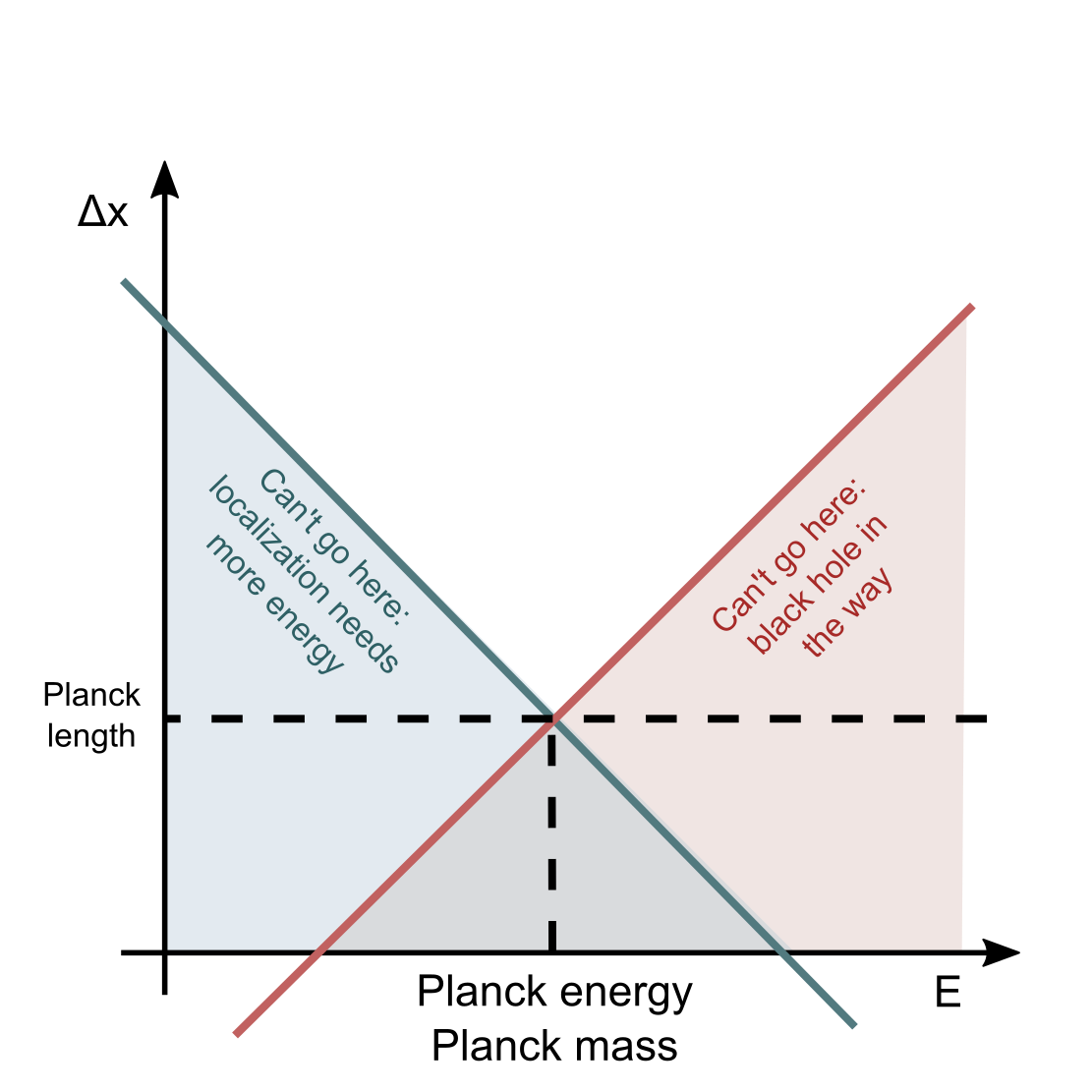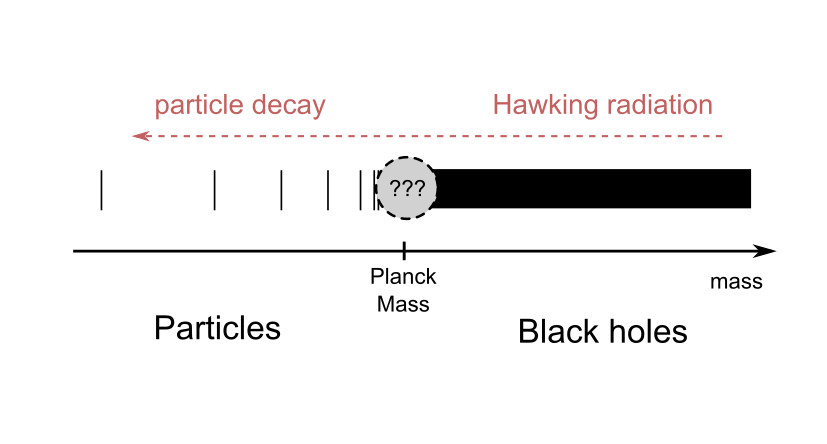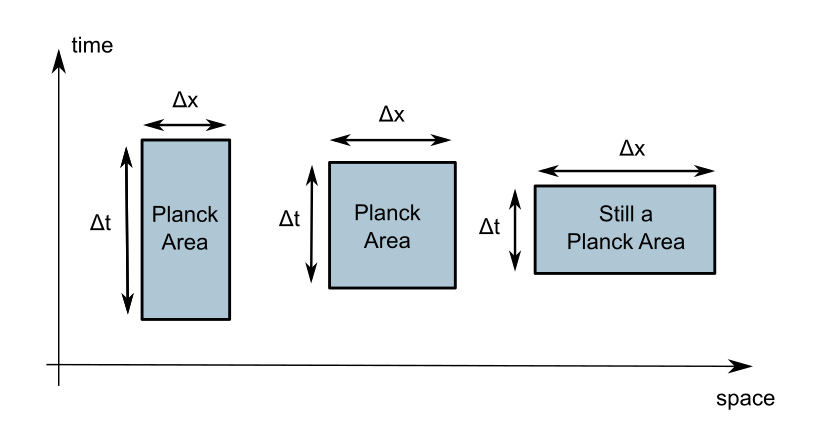# Is the Planck length the minimum possible length?

20 Feb 2017

Don't Betteridge away this question so quickly. It holds more surprises than I thought it did.

There is a massive amount of confusion about this in popular explanations. It traces back to what I believe is the essential issue with pop-physics: everything is fourth-hand, being passed on from layman to layman and degraded with each iteration. In this case I would guess a rough scheme of the story of this misunderstanding went a little bit like this:

• Scientists provide pop-sci with the notion that "the Planck length is the minimum meaningful distance", which is a simplified representation of a correct insight.
• Pop-sci journalists and enthusiast pass the notion on, until it gets deformed into "the Planck length is like a pixel size for the Universe", which is incorrect.
• Scientists notice the mistake and release a vaccine to clear out the misunderstanding: "the Planck length is not like a pixel size for the Universe. It is just the scale where quantum gravity becomes relevant". Which is definitely correct, but...
• The pop-science cycle chews on this until the notion has been transformed into "the Planck length has never been a minimum distance, it is a misconception. It's just a scale where our current theories break down, and there is nothing to suggest you could not reach down to smaller scales". Which sounds reasonable, but it's not correct.

With this rant about popular physics out of the way, let me try to present some hopefully convincing evidence that the facts I claimed are correct are correct, and vice versa.

First, let's clarify we're going to work in orders of magnitude. Factors of 2 or π do not matter,  in fact essentially only the dependence on dimensionful constant will be of interest - in the end there is an overall fuzziness to it from our own ignorance about quantum gravity anyway. So, from the top to the bottom of this article, it's orders of magnitude.

Imagine trying to locate the position of something, an event, or an object, to a small precision $$\Delta X$$. What is the most efficient way to do that? I mean: how do you perform this measurement while disturbing your something as little as possible? There is a lower bound given by relativistic quantum mechanics. We know all objects in quantum mechanics are ultimately de Broglie waves, and are bound by the Heisenberg uncertainty principle:

$$\Delta X \Delta P \gtrsim \hbar$$

meaning the minimum momentum uncertainty that the probe we use to locate our position must be $$\Delta P \sim \hbar / \Delta X$$. Now we throw in relativity. The energy of a relativistic particle is

$$E^2 = m^2 c^4 + P^2 c^2$$

which implies the minimum energy given a certain momentum is obtained for massless particles (or you can also use ultrarelativistic particles like $$m c^2 \ll Pc$$. If you're shooting them at 1Gev, electrons look massless too). In that case, the absolute minimum energy kick we must necessarily give to locate a position to $$\Delta X$$ is

$$\Delta E \sim \frac{\hbar c}{\Delta X}$$

which is of course nothing else than the relationship between the energy and wavelength of a photon (minus or plus an order of magnitude, of course). So, let's use photons, since they're optimal in this sense, and if you want you can imagine using two opposite incident photons, so you don't give any linear momentum to your something, which would be pointlessly confusing.

So this is the famous energy-length scales relationship of relativistic QM, or better a bound: if you are at the energy scale $$E$$, you cannot probe distances smaller than $$L \sim \frac{\hbar c}{E}$$, or if you are probing distances $$L$$, the energy cannot be lower than $$E \sim \frac{\hbar c}{L}$$.

Now, gravity. General relativity generates a different energy-distance bound. If you have a certain energy $$E$$ (or mass $$M = E/c^2$$) available, there is a minimum space you can squeeze it in: the most compact possible object is a black hole. The radius of the black hole is the Schwarzschild radius:

$$r_S \sim \frac{GM}{c^2} = \frac{GE}{c^4}$$

Note the two constants $$c^2/G$$ and $$c^4/G$$, these are respectively the Planck linear mass density and Planck force. We'll talk about these later. For now, it's important to note that the GR bound has a linear, instead of inverse, relationship between space uncertainty and energy. It tells us you that to localize something to a position uncertainty $$\Delta X$$, you can use at most the energy $$E \sim \frac{c^4}{G} \Delta X$$, if you use any more, you create a black hole larger than the precision you want and your measurement is ruined.

Since the bounds we found are power laws, let's place them in a log-log plot where they are straight lines:Blue is the contribution of relativistic quantum mechanics. Red is from general relativity. Sorry if you’re daltonic.

One goes down, one goes up. When you combine the bounds, you get an absolute bound on the position uncertainty. Trusting these bounds, it seems as if there is a minimum length scale where the Compton and Schwarzschild lengths coincide. That's

$$\frac{\hbar c}{E} = \frac{GE}{c^4} \Rightarrow E = \sqrt{\frac{\hbar c^5}{G}}$$

or

$$\lambda_C = r_S = \sqrt{\frac{\hbar G}{c^3}} =: l_P$$

the infamous Planck length. So, within an order of magnitude, you shouldn't be able to probe shorter distances than $$l_P$$. At $$l_P$$, the energy we need to develop is the Planck energy $$E_P = \sqrt{\frac{\hbar c^5}G}\,,$$ and the equivalent mass is the Planck mass $$M_P = E_P/c^2 = \sqrt{\frac{\hbar c}{G}}\,.$$ Note that while the Planck length seems like a lower bound to all physics, the Planck mass or energy are boundaries between different areas of physics. $$M_P$$ is the threshold between particle physics and gravity, being an upper bound on the mass of fundamental particles and a lower bound on the mass of a black hole. As a black hole evaporates down to the Planck mass from Hawking radiation, you would expect it to morph into particle(s) as it jumps this fence.The discrete mass spectrum of particles should morph into the continuous spectrum of black hole masses as the Planck mass is overcome. Some kind of “liminal being” must interpolate in this unknown region between particle and black hole.

Actually, that's not really rigorous because the theories we used to derive the bounds are surely not valid up to the Planck scale. If we use relativistic quantum mechanics and raise the energy up to near the Planck energy, there is an unlimited number of gravitational corrections that join the party as the strength of gravity becomes larger and larger, and these corrections are "uncontrollable" and render the theory useless - the least confusing description of the non-renormalizability of gravity I can manage in one sentence. Conversely, if I start from classical general relativity and lower the mass down to near the Planck mass, higher loop (quantum) corrections appear and the same problem arises. Basically, both quantum field theory and general relativity should be replaced by a consistent theory of quantum gravity, which presents itself in full glory only when you scrape the Planck scale, and otherwise at larger length scales reconstructs quantum field theory and general relativity. In pictures, there is a "small" fuzzy patch we need to cut out from our diagram to account for our ignorance:

Now, none of us here is worthy of witnessing the face of God, but I think we all have some mild assumptions on what it surely does not look like. So while a lack of perfect knowledge of Planck-scale physics precludes us from announcing the Planck length is the minimum length scale (again, within an order of magnitude and certainly not as a "hard" limit) with absolute certainty, we cannot avoid wondering how bizarre things would need to be in quantum gravity for it not to be, and with a precise and scientific notion of bizarreness: the concept of naturalness. Our bound seems to suggest a bubbly or foamy spacetime, evaporating away as we zoom down to the Planck scale, where geometry itself is affected by quantum uncertainties. Note we found something like

$$(\Delta x)^2 \gtrsim l_P^2$$

But we could as well swap $$\Delta x$$ with $$\Delta t/c$$, since we're definitely in the relativistic regime. In fact, instead of talking about Compton wavelength, we could have talked about Compton frequency/period, and we'd have obtained an equivalent-ish but much more eloquent

$$\Delta x \Delta t \gtrsim l_P^2 /c$$

Huh. Time and space seem connected by a sort of uncertainty principle... a space-time uncertainty principle! And just like the familiar position-momentum HUP arises from a commutation relation $$[x,p] \sim \hbar$$, so the possibility arises that space and time do not commute, and the commutator is of order the Planck area:

$$[x,t] \sim l_P^2 / c$$

Or you could do this with two different space dimensions, instead of space and time. In any case, there is a suggestion that the geometry itself of spacetime is non-commutative, or that non-commutative geometry (for what little I understand of it at least) should play a key role in quantum gravity. Anyway, the space-time uncertainty principle is a weaker refinement of our bound and seems to suggest that rather than being impossible to squeeze $$\Delta x$$ below the Planck length, it is indeed possible to probe smaller lengths provided you allow for a large time uncertainty. You could perhaps locate the position of an event as good as you want along a single axis if you are willing to allow a large indeterminacy in the time at which it happens, or in its position along other spatial axes.Uncertainty boxes saturating the space-time uncertainty principle. The Planck area acts as a sort of “minimum area” or quantum of area for spacetime, in a very similar way in which ( \hbar) acts as the quantum of phase space volume in quantum mechanics

And now, we were talking about naturalness... for this fundamental space-time foaminess not to arise, so that we can simply move forward to lower length scales as if nothing happened, would require that all those infinite higher-loop quantum corrections all conspire to cancel this uncertainty to zero, while it would very naturally be around $$l_P^2$$. It requires a huge degree of fine-tuning for no apparent reason. Instead, it reasonable to assume this doesn't happen - it's not a proof, but certainly rephrases the question in a way that makes it clear which possibility is the "standard" one and which one is absurd and exotic. And the only reason that for us at large length scales this foaminess is not visible is pure dimensional analysis!

Still, this picture is very alien compared to the naive "pixely" one. Distances shorter than the Planck scale don't make sense in the sense that the inherent quantumness of spacetime pushes back. It's like trying to squeeze water in your hand only to have it squish out from the sides.

But then, it does not end here. We do have an explicit example of a working theory of quantum gravity in which to verify these deductions more precisely: string theory. In string theory the space-time uncertainty relations arise from the weird property that strings have of stretching and becoming longer when you give them a lot of energy, more precisely

$$\Delta x \sim \frac{l_S^2 }{c\hbar} \Delta E$$

where $$l_s$$ is a constant known as the string length. (Now, the string and Planck lengths are not the same thing, but I'm going to intentionally confuse them since the difference is not essential for this reasoning). The name of the string length comes from the fact that when instead strings have little energy, they don't shrink down to zero size but rather settle on a minimum constant size:

$$\Delta x \sim l_S$$

Now it should be easy to see that the high-energy "stretching" behaviour combined with $$\Delta E \sim \frac{\hbar}{\Delta t}$$ implies the time-space uncertainty:

$$\Delta x \Delta t \gtrsim l_S^2$$

and that the low-energy behaviour instead implies that the string length is the minimum sensible scale:

$$\Delta x \gtrsim l_S$$

Ultimately, the foaminess is interpreted in (perturbative) string theory as due to the fact that strings are extended objects that cannot get smaller than $$\sim l_S$$, and that a localization measurement could not be done with anything else but strings. Or rather, you could: you could use a D-brane (like a D0-brane, a D-particle) to perform a position measurement. This allows you to break the $$\Delta x \sim l_S$$ barrier and measure smaller lengths, but you must still satisfy the space-time uncertainty principle and must therefore move your D-brane very slowly, and let the measurement take a proportionally long time.

This can be done much, much more precisely but I'm not bothering here of course. If you want to read more this is great.

Also, as an aside: string theory provides the "liminal beings" interpolating between particle and black hole! They are... drum roll... strings. A little-excited string is just a particle, in fact a point particle when seen from our infrared, large scale perspective since $$l_S$$ is very small. There is a discrete spectrum of excited states and those are the possible particles. All of them are essentially of size $$l_S$$. As you give it more and more energy you approach the Planck energy and the number of possible states grows vertiginously. (As $$\exp( \text{constant} \cdot \frac{l_S}{\hbar c} \Delta E)$$ actually). The string begins getting longer and longer; as the mass rises above $$M_P$$ the spectrum of states becomes continuous and the string turns into a tangly, warm wooly membrane above a baby horizon, a black hole. This transition can be study pretty explictly.

Be it correct or not in terms of our Universe, the fact that string theory confirms, with a much more specific and quantitative presentation, our hand-wavy expectations about quantum gravity is notable. It is certainly a good sign.

### Addendum: why no numeric values?

Why haven't I mentioned anything about the actual values in SI units of the Planck length, mass...? Sure, the calculations are order-of-magnitude, but at least the orders of magnitude (e.g. $$l_P \sim 10^{-34} m$$) could be interesting to talk about, right? Well, the point is while I think the arguments above about what happens at the Planck scale are true, I don't necessarily believe the Planck scale must be where you would naively think it is based on the calculation above. Let me explain. We found a Planck length which is small if gravity is weak:

$$l_P \sim G^{1/2}$$

However, the strength of gravity that we measure here might not be genuine, it might be a fake. This happens for example if there are extra compact dimensions which are large, that is $$\gg l_P$$. In that case, when you are at small scales, smaller than the compact dimensions, you are in a higher-dimensional (say, (9+1)-dimensional) space with a certain strength of gravity $$G_{(10)}$$ and a "ten-dimensional Planck length"

$${l_P}_{(10)} \sim G_{(10)}^{1/8}$$

(hey, why the eight root? Dimensional analysis!) which is the "true" actual Planck length where all the magic stuff happens. However, as you hop to larger length scales, larger than the compact dimensions, and return to our 4-dimensional Universe, gravity gets an extra weakening. The gravitational constant we measure (the "4-dimensional" big G) is the 10-dimensional one divided by the volume of the 6 extra dimensions

$$G_{(4)} = \frac{G_{(10)}}{V_6}$$

So, our gravity is weaker than normal... which means our $${l_P}_{(4)}$$ calculated from $$G_{(4)}$$ is an incorrect estimate, smaller than the true Planck length $${l_P}_{(10)}$$. Our argument has to be corrected because as dive below the scale of the extra dimensions, it becomes easier to create a black hole.

## All Planck units

The Planck length is a "minimum length", where the expression is to be understood in the sense I attempted to explain above. What about all the other Planck units? Not all of them are necessarily interpretable as bounds; I tried to compile them in a table here with my personal attempt at an interpretation of this type. It's a work in progress.

Planck units are all dimensionful quantities constructible from powers of $$G,c,\hbar$$. I've decided to use units with $$k_B = 1$$ because man, that's one stupid constant, and I'm not going to touch on anything electromagnetic. There is exactly one Planck quantity for a given unit, since the three fundamental constants are independent. Some Planck units only require a subset of the constants, and these are indeed relevant not to general quantum gravity but to some "subtheory" - it should become clear as you scroll down the table.

All of this make sense to within an order of magnitude, of course.

## Newtonian gravity ($$G$$)

$$G$$, units $$M^{-1} L^3 s^{-2}$$, gives the strength of gravity.

## Special relativity ($$c$$)

$$c$$, units $$L T^{-1}$$, Planck speed, maximum possible speed.

## Quantum mechanics ($$\hbar$$)

$$J_P = \hbar$$, units $$M L^2 T^{-2}$$, Planck angular momentum / Planck action, minimum possible angular momentum or action. Phase space volume is "granular" and cells have volume $$\hbar$$.

## Quantum Newtonian gravity / non-relativistic quantum gravity ($$G, \hbar$$)

I found no combination of $$G,\hbar$$ with a clear interpretation.

## Relativistic quantum mechanics ($$\hbar, c$$)

$$\hbar c$$, units $$M L^3 T^{-2} = E L$$, minimum energy per unit inverse length.

## General Relativity ($$G, c$$)

The bounds here are enforced by existence, and occasional inevitability, of black holes.

$$\lambda_P = \frac{c^2}{G}$$, units $$M L^{-1}$$, Planck linear mass density. Maximum possible linear mass density, and that of a black hole. I haven't done the calculation but I think cosmic strings with higher density will fragment into black holes.

$$F_P = \frac{c^4}{G}$$, units $$M L T^{-2}$$, Planck force, maximum possible force in a system at equilibrium and the force between two close black holes. Attempt to produce larger forces will result in enough energy to turn everything into a black hole. Also typical tension of a string.

$$W_P = \frac{c^5}{G}$$, units $$M L^2 T^{-3}$$, Planck power. Maximum power emitted by a black hole merger. Possibly maximum power in some sense I cannot be bothered to think about now.

## Quantum Gravity ($$G, c, \hbar$$)

All possible remaining units combinations can be made with these. The (soft) bounds here are enforced by the non-commutative nature of spacetime and the space-time uncertainty principle.

$$l_P = \sqrt\frac{G\hbar}{c^3}$$, units $$L$$, Planck length, minimum possible length in the sense described before.

$$t_P = \sqrt\frac{G\hbar}{c^5}$$, units $$T$$, Planck time, minimum possible time in the sense described before.

$$l_P^2 = \frac{G\hbar}{c^3}$$, Planck area, whatever, you get these.

$$T_P = E_P = \sqrt\frac{\hbar c^5 }{G}$$, units $$\Theta = E = M L^2 T^{-2}$$, Planck temperature or Planck energy. Maximum possible temperature.

$$M_P = \sqrt\frac{\hbar G}{c^3}$$, units $$M$$, maximum mass of a particle and minimum mass of a black hole.

$$a_P = \sqrt\frac{c^7}{\hbar G}$$, units $$L T^{-2}$$, Planck acceleration, maximum possible acceleration. At this acceleration, a Rindler horizon appears a Planck length away and emits Planck-temperature Unruh radiation; no kind of structure or information can survive without being irreparably scrambled.

Published on 20 Feb 2017